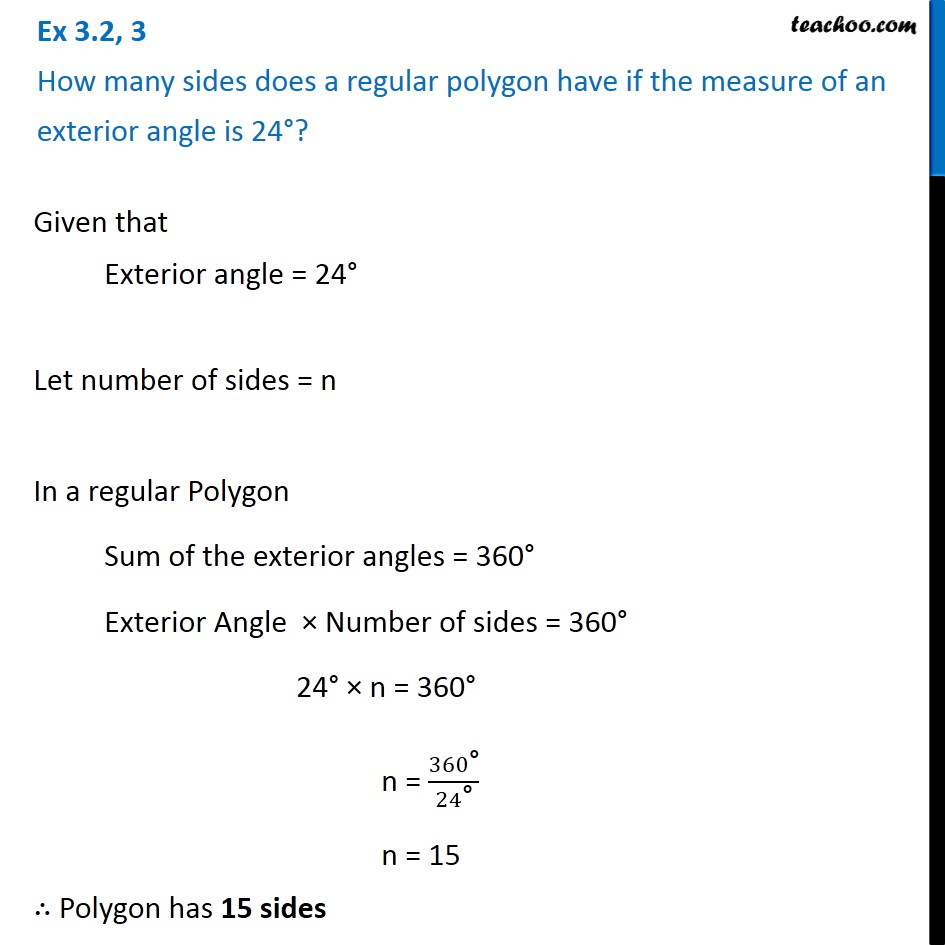Sum of Exterior Angles of PolygonsLearn in your speed, with individual attention - Teachoo Maths 1-on-1 Class

### Transcript

Ex 3.2, 3 How many sides does a regular polygon have if the measure of an exterior angle is 24°? Given that Exterior angle = 24° Let number of sides = n In a regular Polygon Sum of the exterior angles = 360° Exterior Angle × Number of sides = 360° 24° × n = 360° n = 360"°" /24"°" n = 15 ∴ Polygon has 15 sides